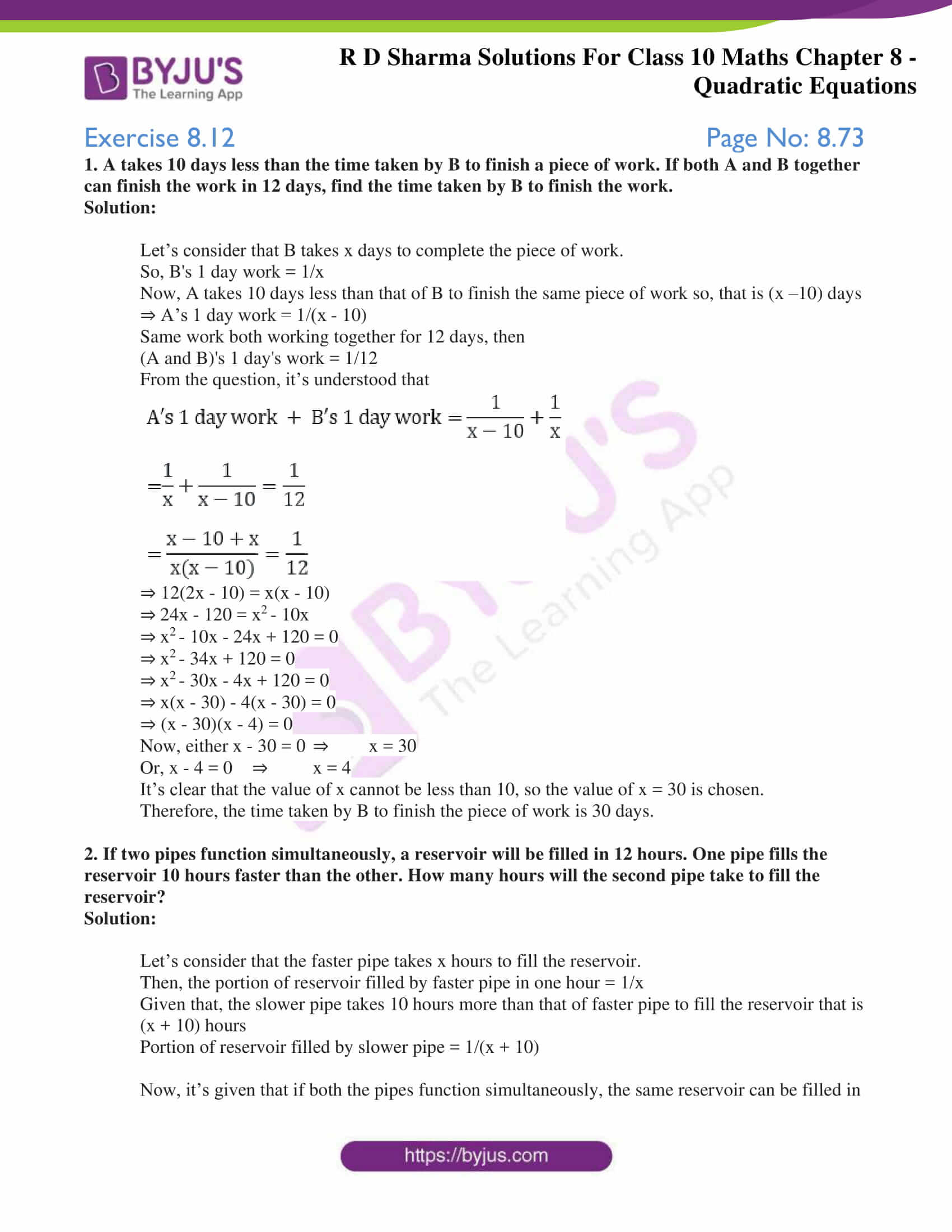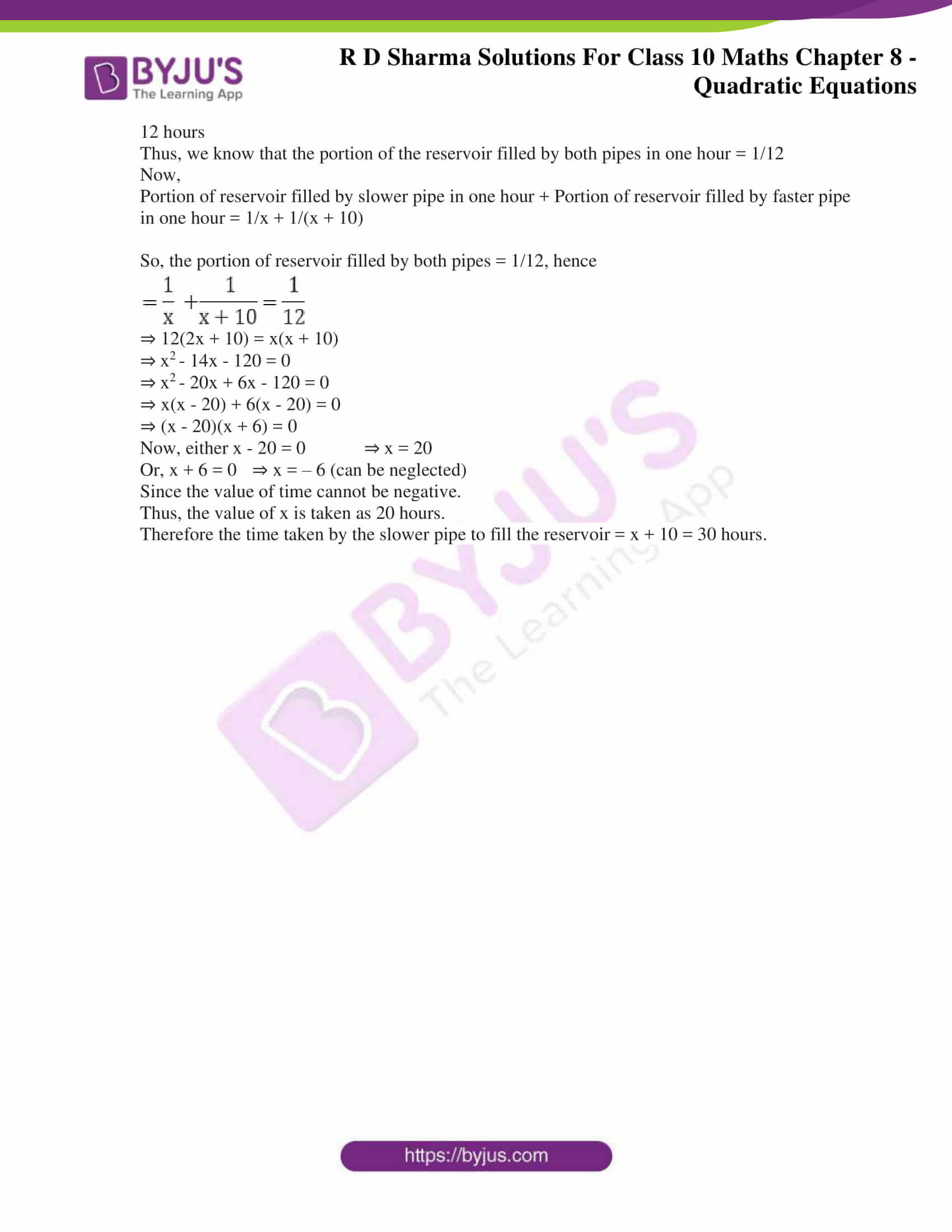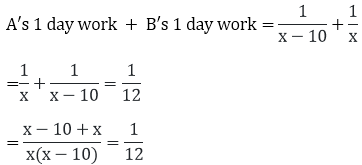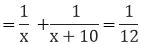# RD Sharma Solutions for Class 10 Maths Chapter 8 Quadratic Equations Exercise 8.12

Out of many applications of quadratic equations, the problems on time and work is an interesting one. So, this exercise briefs problem on this particular application of quadratic equations. Students willing to self assess can refer to RD Sharma Solutions Class 10 to know where they are lacking. Also, the students can access the RD Sharma Solutions for Class 10 Maths Chapter 8 Quadratic Equations Exercise 8.12 PDF given below.### Access RD Sharma Solutions for Class 10 Maths Chapter 8 Quadratic Equations Exercise 8.12

1. A takes 10 days less than the time taken by B to finish a piece of work. If both A and B together can finish the work in 12 days, find the time taken by B to finish the work.

Solution:

Let’s consider that B takes x days to complete the piece of work.

So, B’s 1 day work = 1/x

Now, A takes 10 days less than that of B to finish the same piece of work so, that is (x –10) days

⇒ A’s 1 day work = 1/(x – 10)

Same work both working together for 12 days, then

(A and B)’s 1 day’s work = 1/12

From the question, it’s understood that⇒ 12(2x – 10) = x(x – 10)

⇒ 24x – 120 = x– 10x

⇒ x– 10x – 24x + 120 = 0

⇒ x– 34x + 120 = 0

⇒ x– 30x – 4x + 120 = 0

⇒ x(x – 30) – 4(x – 30) = 0

⇒ (x – 30)(x – 4) = 0

Now, either x – 30 = 0 ⇒ x = 30

Or, x – 4 = 0 ⇒ x = 4

It’s clear that the value of x cannot be less than 10, so the value of x = 30 is chosen.

Therefore, the time taken by B to finish the piece of work is 30 days.

2. If two pipes function simultaneously, a reservoir will be filled in 12 hours. One pipe fills the reservoir 10 hours faster than the other. How many hours will the second pipe take to fill the reservoir?

Solution:

Let’s consider that the faster pipe takes x hours to fill the reservoir.

Then, the portion of reservoir filled by faster pipe in one hour = 1/x

Given that, the slower pipe takes 10 hours more than that of faster pipe to fill the reservoir that is (x + 10) hours

Portion of reservoir filled by slower pipe = 1/(x + 10)

Now, it’s given that if both the pipes function simultaneously, the same reservoir can be filled in 12 hours

Thus, we know that the portion of the reservoir filled by both pipes in one hour = 1/12

Now,

Portion of reservoir filled by slower pipe in one hour + Portion of reservoir filled by faster pipe in one hour = 1/x + 1/(x + 10)

So, the portion of reservoir filled by both pipes = 1/12, hence⇒ 12(2x + 10) = x(x + 10)

⇒ x– 14x – 120 = 0

⇒ x– 20x + 6x – 120 = 0

⇒ x(x – 20) + 6(x – 20) = 0

⇒ (x – 20)(x + 6) = 0

Now, either x – 20 = 0 ⇒ x = 20

Or, x + 6 = 0 ⇒ x = – 6 (can be neglected)

Since the value of time cannot be negative.

Thus, the value of x is taken as 20 hours.

Therefore the time taken by the slower pipe to fill the reservoir = x + 10 = 30 hours.